# 详解最大似然估计（MLE）、最大后验概率估计（MAP），以及贝叶斯公式的理解

$P(A|B) = \frac{P(B|A)P(A)}{P(B)} \tag{1}$

$P(A|B) = \frac{P(B|A)P(A)}{P(B|A)P(A) + P(B|\sim A)P(\sim A)} （∼A表示”非A”）\tag{2}$

——————不行，还得先说似然函数（likelihood function）

$P(x|\theta)$

\begin{aligned} f(x_0 ,\theta) = (1-\theta)\times\theta\times\theta\times\theta\times\theta\times(1-\theta)\times\theta\times\theta\times\theta\times(1-\theta) \\ = \theta ^ 7(1 - \theta)^3 = f(\theta) \end{aligned}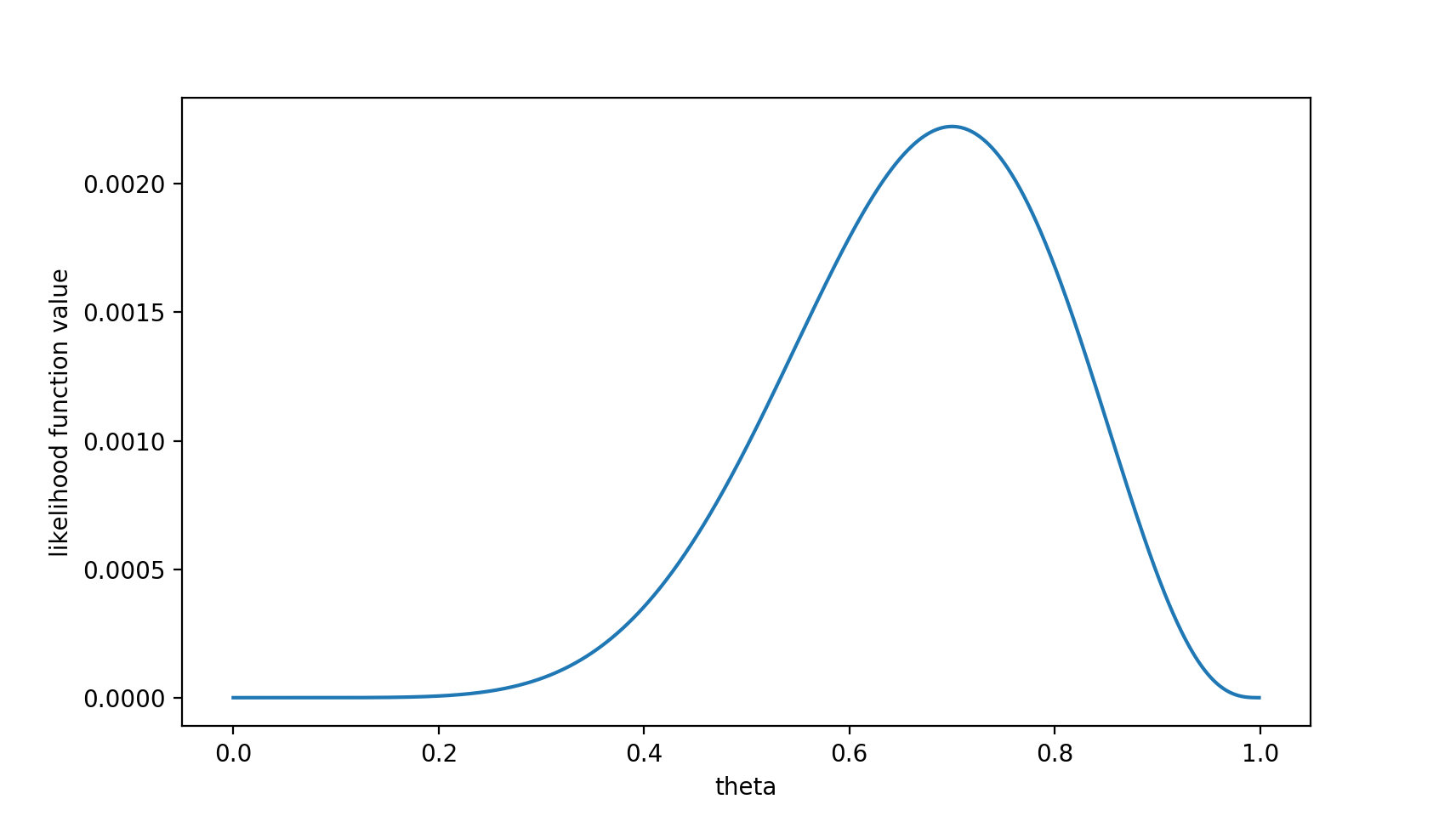MAP其实是在最大化$P(\theta|x_0) = \frac{P(x_0|\theta)P(\theta)}{P(x_0)})$，不过因为$x_0$是确定的（即投出的“反正正正正反正正正反”），$P(x_0)$是一个已知值，所以去掉了分母$P(x_0)$（假设“投10次硬币”是一次实验，实验做了1000次，“反正正正正反正正正反”出现了n次，则$P(x_0)=n/1000$。总之，这是一个可以由数据集得到的值）。最大化$P(θ|x_0)$的意义也很明确，$x_0$已经出现了，要求$θ$取什么值使$P(θ|x_0)$最大。顺带一提，$P(θ|x_0)$即后验概率，这就是“最大后验概率估计”名字的由来。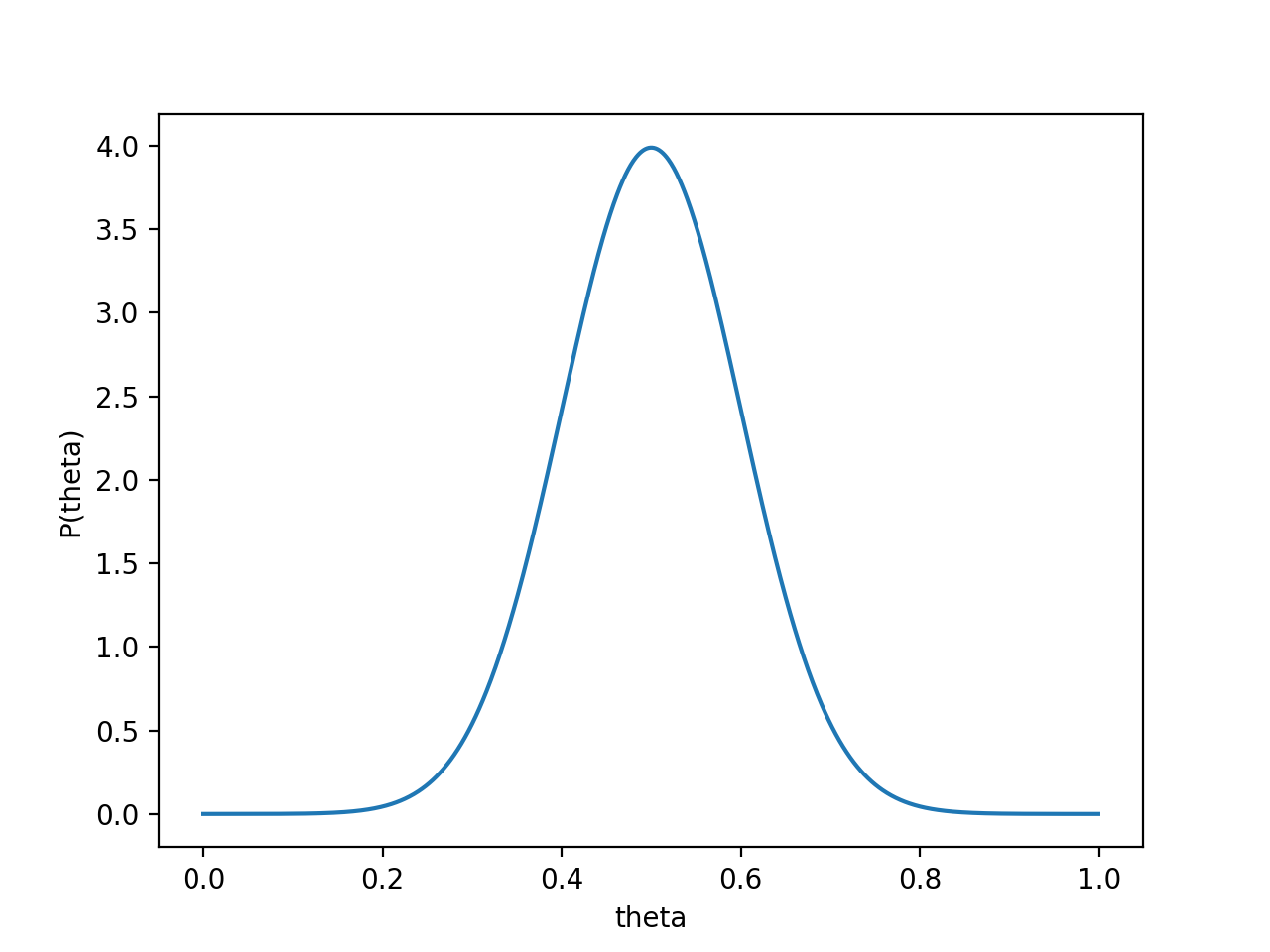$P(x_0|θ)P(θ)$的函数图像为：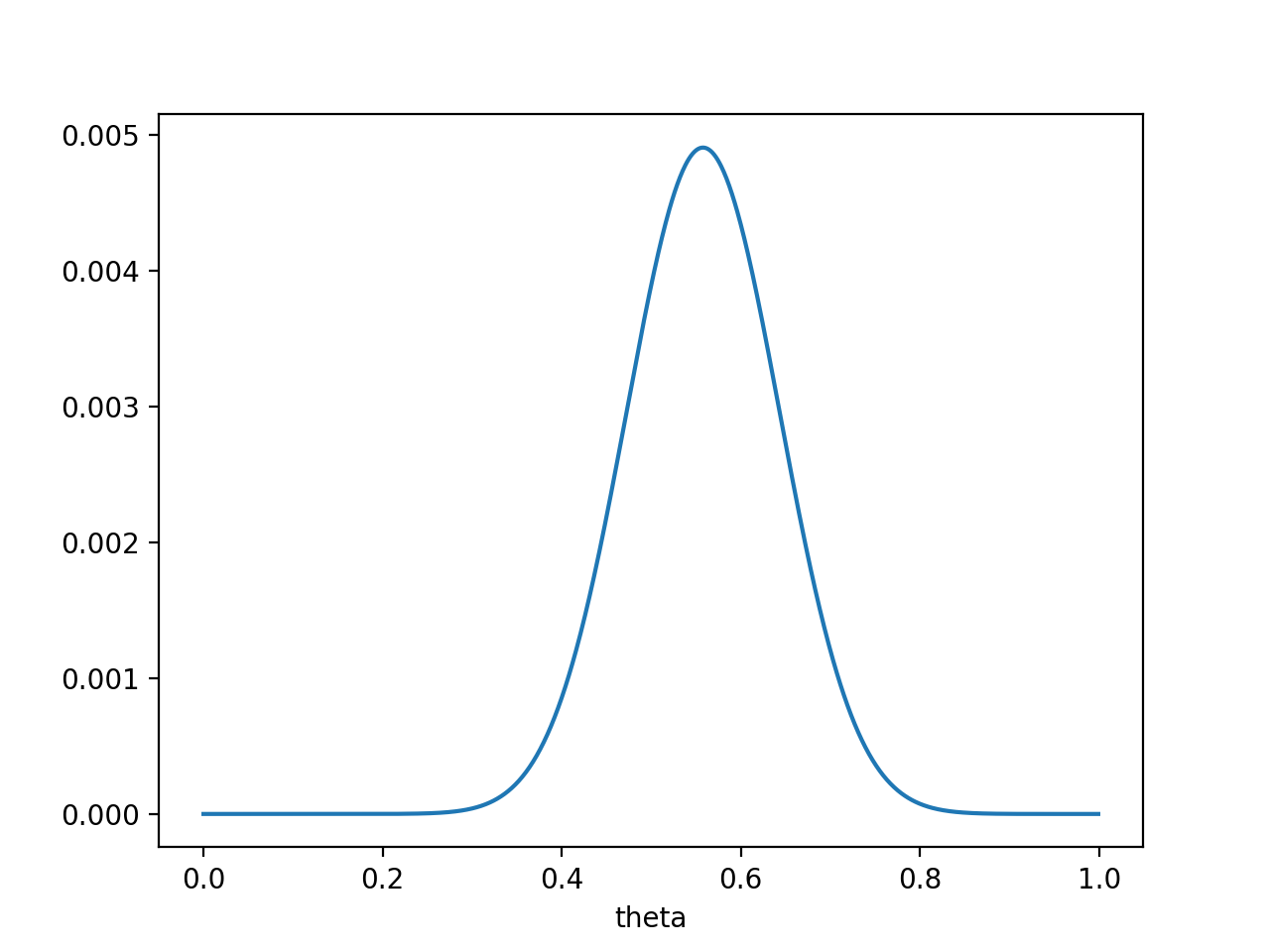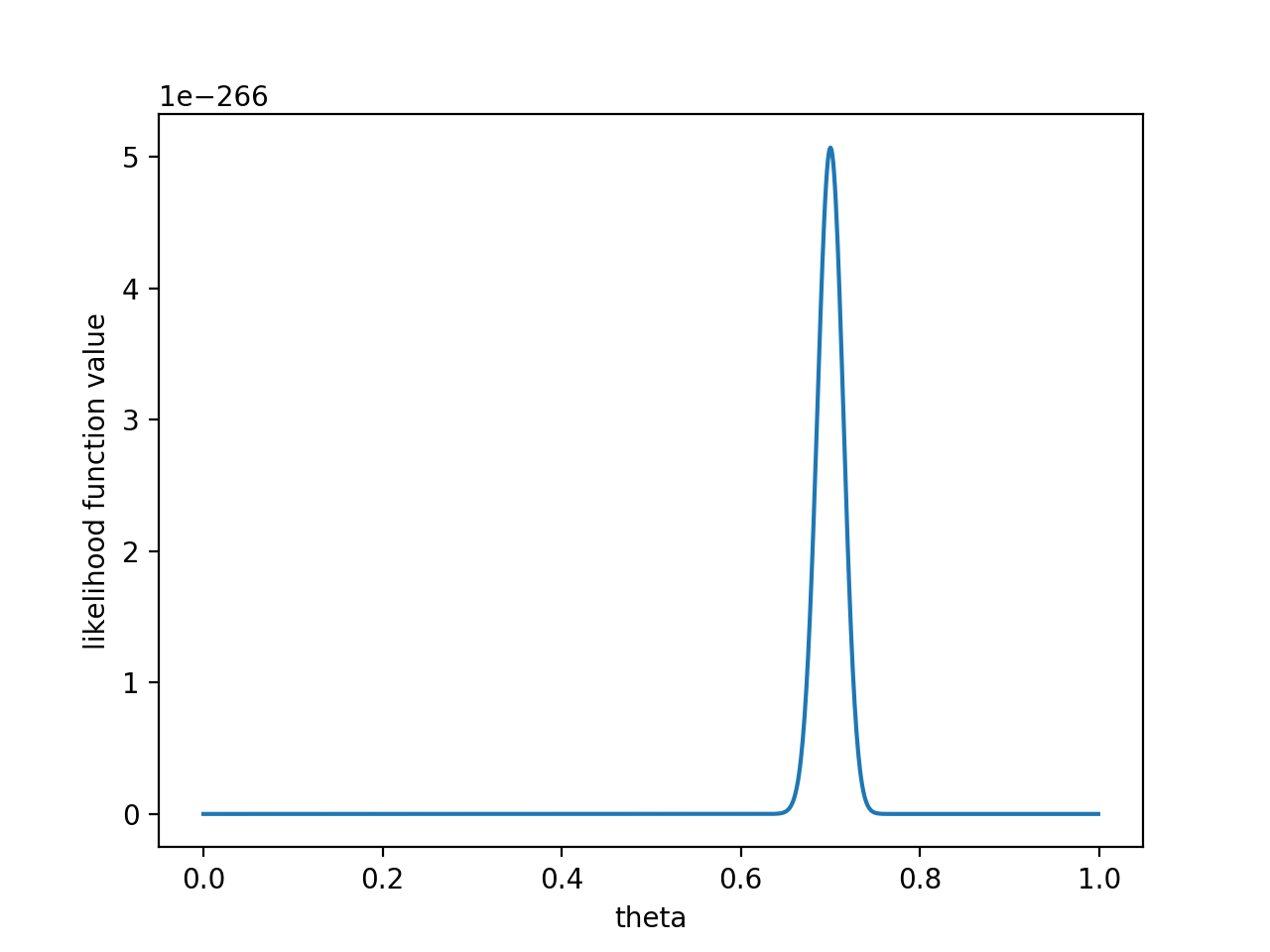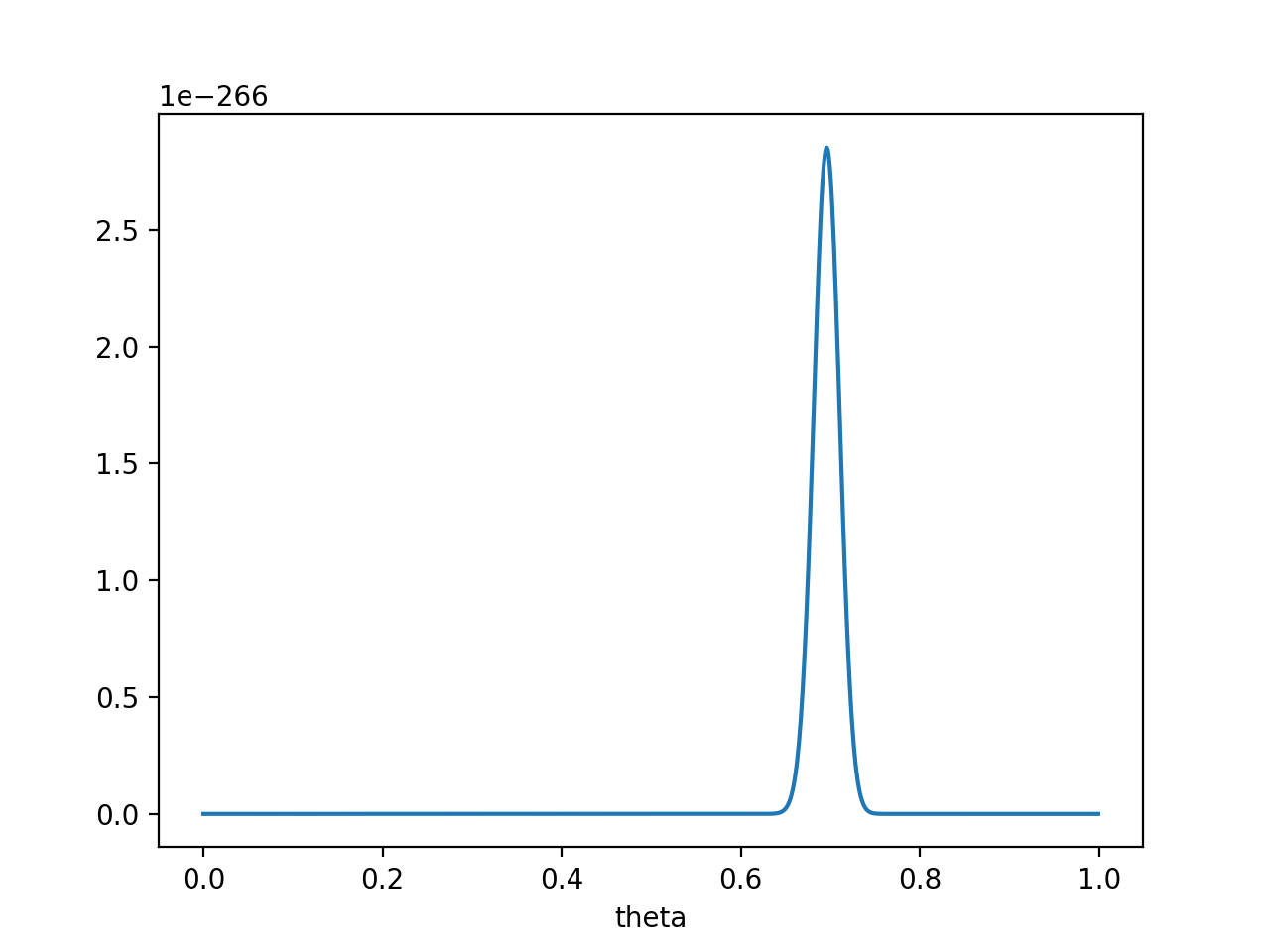$θ=0.696$处，$P(x_0|θ)P(θ)$取得最大值。

PS. 要是遇上了顽固的贝叶斯派，认为$P(θ=0.5)=1$，那就没得玩了。。 无论怎么做实验，使用MAP估计出来都是$θ=0.5$。这也说明，一个合理的先验概率假设是很重要的。（通常，先验概率能从数据中直接分析得到）